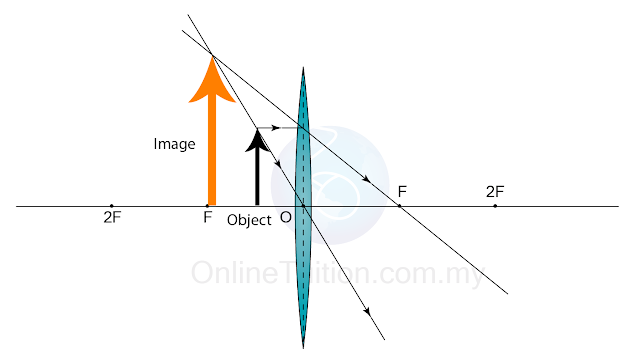# Characteristics of the Image Formed by a Convex Lens

### Characteristics of the Image Formed by a Convex Lens

1. As with a curved mirror, the position and size of an image can be found by drawing a ray diagram.
2. Any two of the following three rays are sufficient to fix the position and size of the image.
3. The characteristics, position and size of the image formed by a convex lens depends on the object distance (u) relative to the focal length (f)

#### Position of Object: u > 2f

Characteristics of the Image: Real, inverted, diminish
Distance of image: v < 2f

#### Position of Object: u = 2f

Characteristics of the Image: Real, inverted, same size
Distance of image: v = 2f

#### Position of Object: f < u < 2f

Characteristics of the Image: Real, inverted, magnified
Distance of image: v > 2f

#### Position of Object: u = f

Characteristics of the Image: -
Distance of image: At infinity

#### Position of Object: u < 2Characteristics of the Image: Virtual, uprigh, magnified
Position of image: at the same side of the object